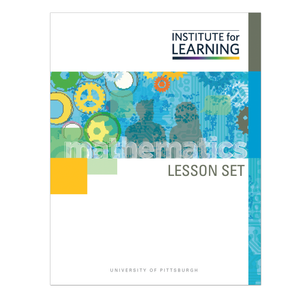# A Focus on Compare Situations (Grade 2)

Regular price \$6.25 \$0.00 Unit price per

This lesson set focuses on compare situations with an unknown difference. In this set, students grapple with the relationship between subtraction and unknown addend addition as they use both operations to compare and determine the difference between amounts. Students also consider why both the operation of subtraction and the operation of addition with an unknown addend can be used when making comparisons. The variety of contexts for story situations has been purposefully designed to provide opportunities for students to compare sets of items, heights, lengths, and distances traveled. Students will:

• solve “compare” story situations with an unknown difference involving sets of items, lengths, heights, and distance traveled and discuss attributes of “compare” story situations;
• discuss the relationship between subtraction and unknown addend addition when determining a comparison situation;
• use strategies to solve compare situations:
• adding on to the small set to equal the larger set; and
• subtracting by removing aligned parts to determine the difference;
• use strategies to solve subtraction equations:
• regrouping a ten into ones in order to subtract; and
• making an easier number to subtract and adjusting
• select and use tools to make sense of situations (base ten blocks, diagrams, and number lines); and
• interpret and make observations about data represented in bar graphs.

Standards: 2.OA.A.1, 2.NBT.B.7, 2.MD.B.5